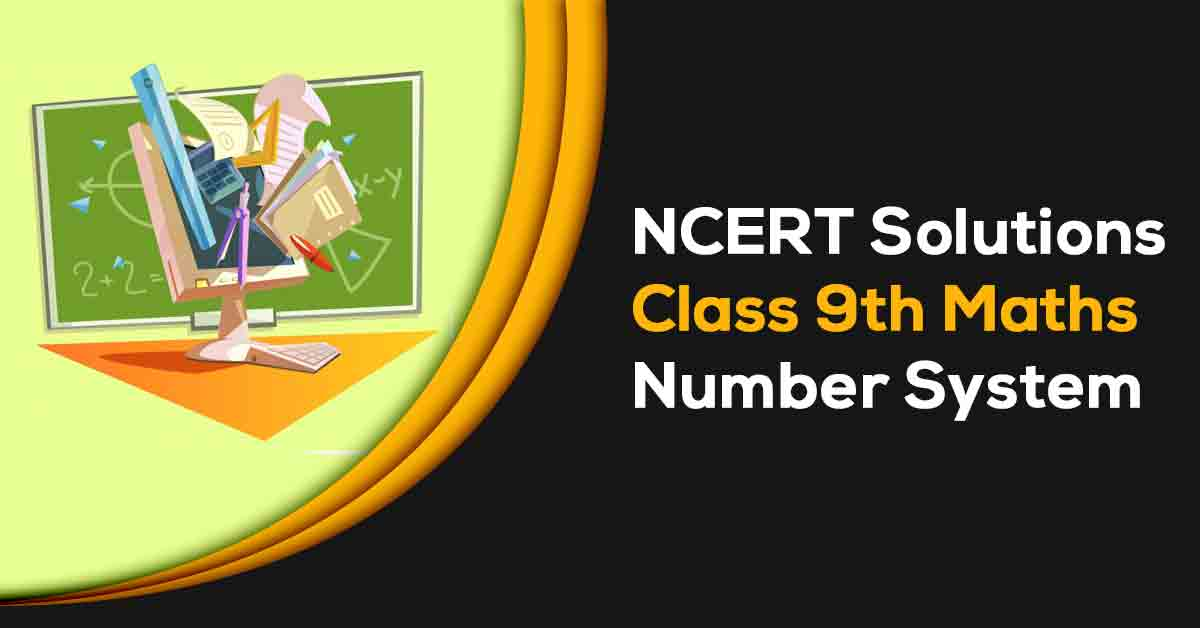Class 9th Math Number System NCERT Solution CBSE 2023

# NCERT Solutions For Class 9 Maths Chapter 1

## NCERT Solutions For Class 9 Maths Chapter 1 Number System  - PDF DownloadStudents require NCERT Solutions for Class 9 Maths Chapter 1 Number System for better exam preparation.  In this chapter, we will learn that a number is called a rational number it is the form of p/q where if both p and q are integers and q is not equal to 0. A number is called an irrational number if it cannot be written in the form of p/q where if both p and q are integers and q is not equal to 0.

These CBSE NCERT Solutions for Class 9 maths chapter 1 Number System will help you to practice all the topics in the chapter with ease and score maximum marks.

What is Number System?

The Set of natural number is called number system. For example: {1,2,3,4,5,...N}